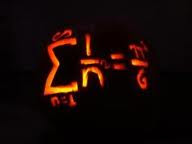# Top 5 Hard Math PuzzlesTop 5 Hard Math Puzzles

Hard Math Puzzles 1 : Brain Twister Puzzle
Difficulty ★★★★☆     Popularity ★★★★★

2+3=8,
3+7=27,
4+5=32,
5+8=60,
6+7=72,
7+8=??

Solve it?

==============================================

Hard Math Puzzles 2 : Weighing Balance Puzzle
Difficulty ★★★★☆     Popularity ★★★★☆

You can place weights on both side of weighing balance and you need to measure all weights between 1 and 1000. For example if you have weights 1 and 3,now you can measure 1,3 and 4 like earlier case, and also you can measure 2,by placing 3 on one side and 1 on the side which contain the substance to be weighed. So question again is how many minimum weights and of what denominations you need to measure all weights from 1kg to 1000kg.

==============================================

Hard Math Puzzles 3 : Hard Maths Puzzle
Difficulty ★★★★☆     Popularity ★★★★☆

A high school has a strange principal. On the first day, he has his students perform an odd opening day ceremony:

There are one thousand lockers and one thousand students in the school. The principal asks the first student to go to every locker and open it. Then he has the second student go to every second locker and close it. The third goes to every third locker and, if it is closed, he opens it, and if it is open, he closes it. The fourth student does this to every fourth locker, and so on. After the process is completed with the thousandth student, how many lockers are open?

==============================================

Hard Math Puzzles 4 : Maths Puzzle
Difficulty ★★★★☆     Popularity ★★★☆☆

How can I get the answer 24 by only using the numbers 8,8,3,3.

You can use the main signs add, subtract multiply and divide.

==============================================

Hard Math Puzzles 5 : December Maths Puzzle
Difficulty ★★★★☆     Popularity ★★☆☆☆

I am thinking of a 6-digit number. The sum of the digits is 43.

And only two of the following three statements about the number are true:

(1) it's a square number,
(2) it's a cube number, and
(3) the number is under 500000.